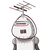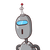# There are 5 kg of potatoes and 3 kg of tomatoes in a bag. What is the total weight of two?​

There are 5 kg of potatoes and 3 kg of tomatoes in a bag. What is the total weight of two?​

### 2 thoughts on “There are 5 kg of potatoes and 3 kg of tomatoes in a bag. What is the total weight of two?​”

1.8kg

Step-by-step explanation:

5kg+3kg=8kg

2.